• 如何求First集与Follow集（超详细）
万次阅读 多人点赞
2020-12-23 18:16:37

## 如何求First集与Follow集

已知文法如下，求First集与Follow集

## First集：

1. 第一次查看所有式子

2. 首先看第一个式子： E → T E ′ E{\rightarrow} TE' ，候选式中未包含终结符，暂时无法得出结果

当前First集：

First(E) = { }
First(E ′ ' ) = { }
First(T) = { }
First(T ′ ' ) = { }
First(F) = { }

3. 第二个式子： E ′ → + T E ′ ∣ ε E'{\rightarrow}+TE'|\varepsilon ，可以得到其所有候选式的串首终结符： +、 ε {\varepsilon} ，将其加入到First(E ′ ' )中

当前First集：

First(E) = { }
First(E ′ ' ) = {+， ε {\varepsilon} }
First(T) = { }
First(T ′ ' ) = { }
First(F) = { }

4. 第三个式子同 1，First集无变化

5. 第四个式子同 2，将 *、 ε {\varepsilon} 加入到First(T ′ ' )中

当前First集：

First(E) = { }
First(E ′ ' ) = {+， ε {\varepsilon} }
First(T) = { }
First(T ′ ' ) = { *， ε {\varepsilon} }
First(F) = { }

6. 第五个式子： F → ( E ) ∣ i F{\rightarrow}(E)|i ，将 （、i 加入到First(F)中

当前First集：

First(E) = { }
First(E ′ ' ) = {+， ε {\varepsilon} }
First(T) = { }
First(T ′ ' ) = { *， ε {\varepsilon} }
First(F) = { (，i}

7. 第二次查看所有式子

8. 第一个式子可得，First(T) 是First(E) ,但First(T)是空的，故无变化

[注：参照书本58页第三行②，若有 X → Y X{\rightarrow}Y , Y Y 是非终结符，就把First(Y)中非 ε {\varepsilon} 元素加入到First(X)中]

9. 第二个式子不能得到新的结果，下一步

10. 第三个式子可得，First(F)是First(T),故将 （ ，i 加入到First(T)
当前First集：

First(E) = { }
First(E ′ ' ) = {+， ε {\varepsilon} }
First(T) = { (，i }
First(T ′ ' ) = { *， ε {\varepsilon} }
First(F) = { (，i }

11. 后续式子均不能得到新的结果，下一步

12. 第三次查看所有式子

13. 第一个式子得，First(T) 是First(E)，故First集产生变化

当前First集：

First(E) = {(，i }
First(E ′ ' ) = {+， ε {\varepsilon} }
First(T) = { (，i }
First(T ′ ' ) = { *， ε {\varepsilon} }
First(F) = { (，i }

14. 后续式子均无法得到新的结果，求First集完毕

15. 注：理论上每次First集产生变化就要重新查看一遍所有式子，这里为了方便进行了简写，实际做题时可以按照变化一次重新求一次

## Follow集：

求Follow集是在已经求出First集的基础上进行

1. 将#置入Follow(文法开始符号)，即Follow(E)

当前Follow集：

Follow(E) = { # }
Follow(E ′ ' ) = { }
Follow(T) = { }
Follow(T ′ ' ) = { }
Follow(F) = { }

2. 第一次查看所有式子

3. E → T E ′ E{\rightarrow} TE' 可得

• Follow(E)可以加入到Follow(E ′ ' )，将 # 置入Follow(E ′ ' )

• Follow(E)可以加入到Follow(T)，将 # 置入Follow(T)

[注：因为E ′ ' 可能为 ε {\varepsilon} ]，

• First(E ′ ' )中非 ε {\varepsilon} 元素可以加入到Follow(T)中，将 + 置入Follow(T)

当前Follow集：

Follow(E) = { # }
Follow(E ′ ' ) = { # }
Follow(T) = { #，+ }
Follow(T ′ ' ) = { }
Follow(F) = { }

4. E ′ → + T E ′ ∣ ε E'{\rightarrow}+TE'|\varepsilon 可得

• Follow(E ′ ' )可以加入到Follow(T)，无变化
5. T → F T ′ T{\rightarrow}FT' 可得

• Follow(T)可以加入到Follow(T ′ ' )，将 #、+ 置入Follow(T ′ ' )

• Follow(T)可以加入到Follow(F)，将 #、+ 置入Follow(F)

• First(T ′ ' )中非 ε {\varepsilon} 元素可以加入到Follow(F)中，将 * 置入Follow(F)

当前Follow集：

Follow(E) = { # }
Follow(E ′ ' ) = { # }
Follow(T) = { #，+ }
Follow(T ′ ' ) = { #，+ }
Follow(F) = {#，+ ，* }

6. T ′ → ∗ F T ′ ∣ ε T'{\rightarrow}*FT'|\varepsilon 可得

• Follow(T ′ ' )可以加入到Follow(F)，无变化
1. F → ( E ) ∣ i F{\rightarrow}(E)|i 可得

• 非终结符E后有一个终结符 ）,故将 ） 置入Follow(E)

当前Follow集：

Follow(E) = { #，） }
Follow(E ′ ' ) = { # }
Follow(T) = { #，+ }
Follow(T ′ ' ) = { #，+ }
Follow(F) = {#，+ ，* }

2. 第二次查看所有式子

3. E → T E ′ E{\rightarrow} TE' 可得

• Follow(E)可以加入到Follow(E ′ ' )，将 ） 置入Follow(E ′ ' )

• Follow(E)可以加入到Follow(T)，将 ） 置入Follow(T)

• First(E ′ ' )中非 ε {\varepsilon} 元素可以加入到Follow(T)中，已有+，Follow(T)无变化

当前Follow集：

Follow(E) = { #，） }
Follow(E ′ ' ) = { # ，） }
Follow(T) = { #，+ ，） }
Follow(T ′ ' ) = { #，+ }
Follow(F) = {#，+ ，* }

4. E ′ → + T E ′ ∣ ε E'{\rightarrow}+TE'|\varepsilon 可得

• Follow(E ′ ' )可以加入到Follow(T)，无变化
1. T → F T ′ T{\rightarrow}FT' 可以得到
• Follow(T)可以加入到Follow(T ′ ' )，将 ） 置入Follow(T ′ ' )

• Follow(T)可以加入到Follow(F)，将 ）置入Follow(F)

• First(T ′ ' )中非 ε {\varepsilon} 元素可以加入到Follow(F)中，已有*，Follow(F)无变化

当前Follow集：

Follow(E) = { #，） }
Follow(E ′ ' ) = { # ，） }
Follow(T) = { #，+ ，） }
Follow(T ′ ' ) = { #，+，） }
Follow(F) = {#，+ ，*，） }

1. T ′ → ∗ F T ′ ∣ ε T'{\rightarrow}*FT'|\varepsilon 可得
• Follow(T ′ ' )可以加入到Follow(F)，无变化
1. F → ( E ) ∣ i F{\rightarrow}(E)|i 并不能得到新的结果
2. 再次查看所有式子，均不能产生新的变化，求Follow集完毕
3. 注：理论上每次Follow集产生变化就要重新查看一遍所有式子，这里为了方便进行了简写，实际做题时可以按照变化一次重新求一次

## 那么在已知First集和Follow集的情况下如何构造LL1分析表呢

### First集与Follow集：

First(E) = {(，i }
First(E ′ ' ) = {+， ε {\varepsilon} }
First(T) = { (，i }
First(T ′ ' ) = { *， ε {\varepsilon} }
First(F) = { (，i }

Follow(E) = { #，） }
Follow(E ′ ' ) = { # ，） }
Follow(T) = { #，+ ，） }
Follow(T ′ ' ) = { #，+，） }
Follow(F) = {#，+ ，*，） }

### LL1分析表：

第一列为非终结符，第一行为终结符

i+*(#
EE遇到 i,i ϵ { \epsilon} First(E),且E只有一个候选式，填入 E → T E ′ E{\rightarrow} TE' E遇到(,( ϵ { \epsilon} First(E),且E只有一个候选式，填入 E → T E ′ E{\rightarrow} TE'
E’+ ϵ { \epsilon} First(E ′ ' )，在E’的候选式中选择+开头的， 填入 E ′ → + T E ′ E'{\rightarrow}+TE' First(E ′ ' )中含有 ε {\varepsilon} ，故E’遇到Follow(E ′ ' )中的终结符时均填入 E ′ → ε E'{\rightarrow} {\varepsilon} E ′ → ε E'{\rightarrow} {\varepsilon}
T T → F T ′ T{\rightarrow}FT' T → F T ′ T{\rightarrow}FT'
T’ T ′ → ε T'{\rightarrow} {\varepsilon} T ′ → ∗ F T ′ T'{\rightarrow}*FT' T ′ → ε T'{\rightarrow} {\varepsilon} T ′ → ε T'{\rightarrow} {\varepsilon}
F F → i F{\rightarrow}i F → ( E ) F{\rightarrow}(E)

注：大写字母表示非终结符，小写字母与符号表示终结符

## 总结：

1. 当非终结符遇到其First集中的终结符时填入相应候选式
2. 当非终结符的First集中含有 ε {\varepsilon} 元素时，遇到其Follow集中的终结符时填入 非终结符 → ε {\rightarrow} {\varepsilon}

如有错误欢迎指正

更多相关内容
• 输入任意的上下文无关文法,输出所输入的上下文无关文法一切非终结符的first集合和follow集合 输入任意的上下文无关文法,输出所输入的上下文无关文法一切非终结符的first集合和follow集合
• 给定文法，构造FIRST集、FOLLOW集的构造的C代码和我个人的实验报告
• 构造预测分析表 源程序 #include<stdlib.h> #include<stdio.h> #include<string.h> /*/ int count=0; /* 分解的产生式的个数 */ int number; /* 所有终结符和非终结符的总数 */ char start; /* 开始符号 */ char ...
• 博文链接：https://zpchen.iteye.com/blog/208947
• ## FIRST集

千次阅读 多人点赞 2019-10-23 00:51:52
FIRST集 1.什么是FIRST集？ 一个符号的FIRST集表示这个符号可以推导出的句子的首符号的集合。 举个例子：A→aa|bb|cc。a,b,c是终结符，则FIRST(A)={a,b,c} 通过上面的定义解释一下，符号A可以推导出三个句子{aa,bb,...

# FIRST集

### 1.什么是FIRST集？

一个符号的FIRST集表示一个符号或句型可以推导出的句子的首符号的集合。

举个例子：A→aa|bb|cc。a,b,c是终结符，则FIRST(A)={a,b,c}

通过上面的定义解释一下，符号A可以推导出三个句子{aa,bb,cc}，那么，这三个句子的首符号集合FIRST(A)={a,b,c}。

## 2.FIRST集求法

再给出最终结论之前，首先看以下的几种情况

### 求一个终结符号的FIRST集

终结符号经过推导（0步推导）后，推导出自己本身，所以如果a是一个终结符号，则FIRST(a)={a}

### 可以推导出空串的非终结符号的FIRST集

如果一个非终结符号可以推导出空串，则有以下两种情况：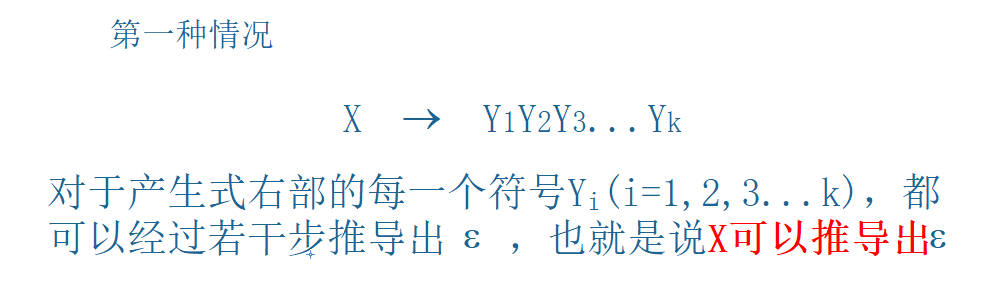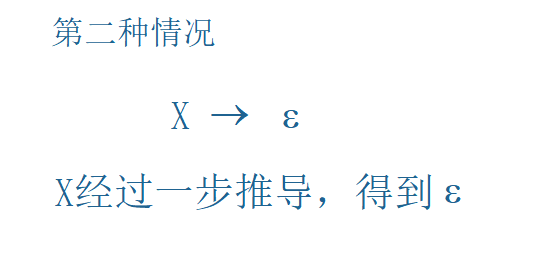对于经过若干步可以推导出空串的非终结符号，有以下结论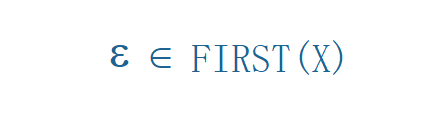### 不能推导出空串的非终结符号的FIRST集

如果一个非终结符号不能推导出空串，它可以被形式的描述为：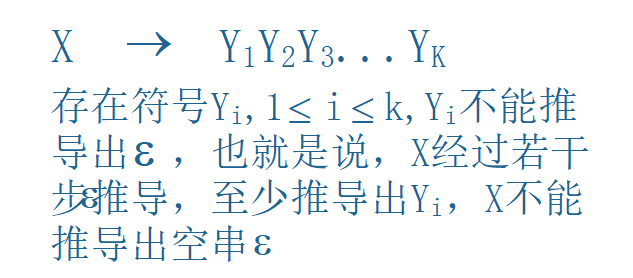对于上面的情况，首先FIRST(Y1)-{ε}(这里减去{ε}的原因下面说)是FIRST(X)的一个子集，因为经过推导之后，用产生式的右部（多个Y）替换了X，则第一个字符就是Y1，所以，FIRST(Y1)-{ε}是FIRST(X)的一个子集（或者说，将FIRST(Y1)-{ε}加入FIRST(X)）。

减去{ε}的原因：如果Y1可以推导出ε，则ε属于FIRST(Y1)，但是仅仅Y1可以推导出空串，还不足以说明X可以推导出空串，只有X可以推导出ε，才可以将ε加入FIRST集（上面讨论的情况）。Y2可能不能推导出空串，所以X就不能推导出空串。

举个例子：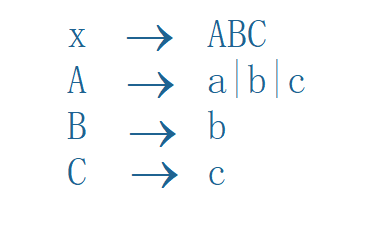如上图的例子，我们可以看到，我们上面讨论的Y1就是A，所以，FIRST(A)-{ε}是FIRST(X)的一个子集（或者说将FIRST(A)-{ε}加入FIRST(X)），因为X只有一条产生式，这个产生式有这一条“线索”，因此分析到这里，就分析结束了，也就是说FIRST(X)=FIRST(A)-{ε}。

所以，问题变成了求FIRST(A)，这里，A有三个产生式，分别推导出终结符号a，b，c。也就是说，对于每一个产生式，Y1分别为a，b，c。FIRST(a)-{ε}，FIRST(b)-{ε}，FIRST( c)-{ε}都是FIRST(A)的子集，也就是说，FIRST(A)=FIRST(a)-{ε}+FIRST(b)-{ε}+FIRST( c)-{ε}={a,b,c}（终结符号的FIRST集就是它自己构成的集合，我们上面讨论过的）

继续分析，上面的情况，我们忽略了Y1可能推导出空串的情况。也就是说，如果将文法做一下修改，变成如下分文法：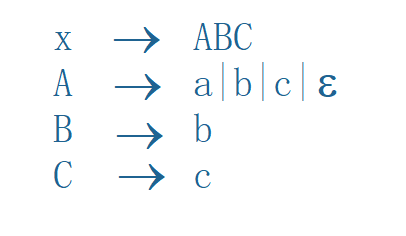想一下，如果X在推导过程中，A使用了空串产生式，那么，B就变成了第一个符号，因此FIRST(B)-{ε}也应该是FIRST(X)的子集。也就是说，如果Y1有空串产生式，则Y2的FIRST集-{ε}是FIRST(X)的子集。

继续进行归纳，如果Y1和Y2都有空串产生式，再将文法修改一下：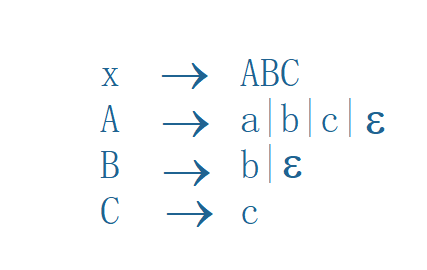如果在X推导过程中，A和B都使用空串产生式，则C就变成了第一个符号，因此FIRST( C)-{ε}也应该是FIRST(X)的子集。也就是说，如果Y1，Y2有空串产生式，则Y3的FIRST集-{ε}是FIRST(X)的子集。

通过归纳，我们可以得到结论，设产生式X→Y1Y2Y3…Yk。则，如果Y1Y2Y3…Yi-1可以推导出空串，也就是说从Y1到Yi，每一个符号都能推导出空串，则Yi的FIRST集-{ε}是FIRST(X)的子集。Y1，Y2…Yi-1这些符号不使用空串产生式时，它们中的每一个都可能成为第一个符号，它们的FIRST集-{ε}都是FIRST(X)的子集。也就是说，对于这种情况，FIRST(X)=FIRST(Y1)-{ε}+FIRST(Y2)-{ε}+…+FIRST(Yi)-{ε}

当然，如果Y1Y2Y3…Yk可以推导出空串，也就是X推导出了空串，这就变成了我们上面讨论的情况。

## 3.总结

1. 如果X是终结符号，则FIRST(X)={X}。
2. 如果X是非终结符号，且X可以推导出ε，则将ε加入FIRST(X)。
3. X→Y1Y2Y3…Yk，若ε∈FIRST(Y1Y2Y3…Yi-1)（i＜k）,则将（FIRST(Y1)-{ε}+FIRST(Y2)-{ε}+…+FIRST(Yi)-{ε}）加入FIRST(X)，否则，将（FIRST(Y1)-{ε}）加入FIRST(X)。如果Y1Y2Y3…Yk可以推导出空串，则就变成了情况2。
4. 补充一条规则，X→Y1Y2Y3…Yk，FIRST(X)⊇FIRST(Y1Y2Y3…Yk)

### 总结方法

如果要求FIRST(X)，首先找到X在左部的产生式，然后对这些产生式应用上面的规则，每应用一次规则，会计算出一个FIRST集的子集，将这些子集加入FIRST(X)。

需要应用两次规则的产生式：对于X→Y1Y2Y3…Yk，如果Y1Y2Y3…Yk可以推导出ε，则就说明X可以推导出ε，因此，就可以利用规则2和规则3，也就是两条规则

举个例子：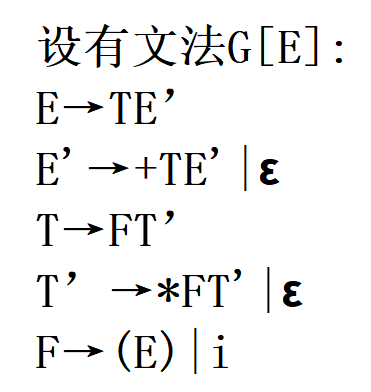求FIRST(E)，首先，找到E在左部的产生式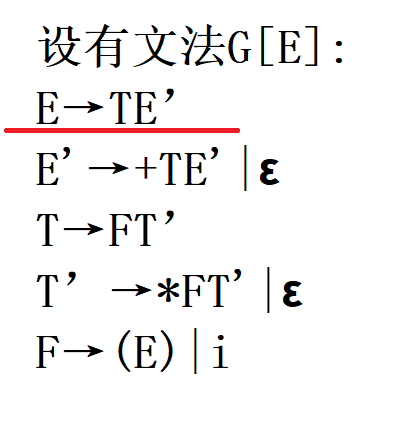可以看到，产生式的形式属于，X→Y1Y2Y3…Yk，首先，将FIRST(T)-{ε}加入FIRST(X)。（应用第三条规则，因为T不能推导出空串，所以不需要讨论E’

问题又变成了求FIRST(T)，找到T在左部的产生式，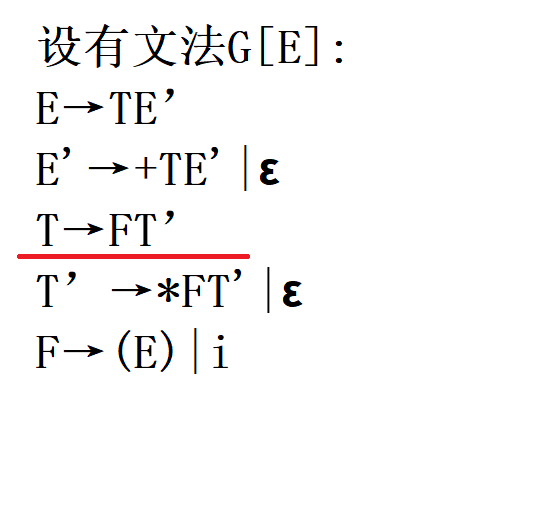同理，将FIRST(T)=FIRST(F)-{ε}（同样，因为F不能推导出ε，而且这里只有一条产生式，且只用了一次规则，所以，可以确定FIRST(T)=FIRST(F)-{ε}）

因此，又要先求FIRST(F)
找到F在产生式左部的产生式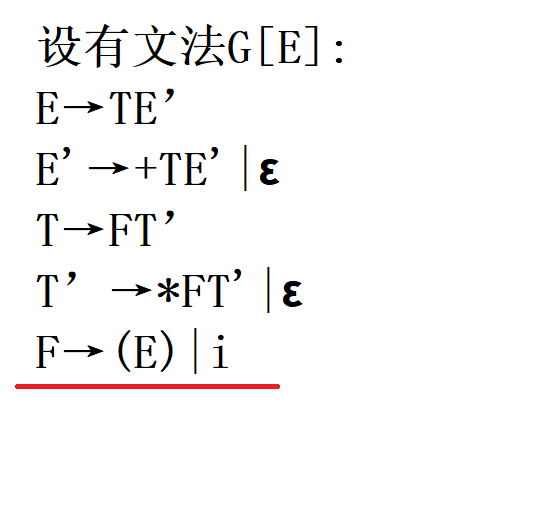可以看到，F在左部的产生式有两个，因此，FIRST(F)=FIRST(()-{ε}+FIRST(i)-{ε}={(, i}

FIRST(T) = FIRST(F)-{ε} = {(, i}
FIRST(E) = FIRST(E)-{ε} = {(, i}

经过上面的分析，我们得到FIRST(E)=FIRST(T)=FIRST(F)={(, i}

接下来分析稍复杂的FIRST(E’)，还是那样，需要找到E’在产生式左部的产生式

有两个产生式，一个是空串产生式，应用规则3；另一个应用规则3，再将这两个结果求并集即可，FIRST(E’)={ε}+(FIRST(+)-{ε})={ε, +}

同理，FIRST(T’)={ε, *}

FIRST(E’T’)=？ 句型E’T’是符合应用两次规则的句型。
第一次应用规则：应用规则3，(FIRST(E’)-{ε}+FIRST(T’)-{ε})是FIRST(E’T’)的子集。

第二次应用规则：应用规则2，又因为E’T’可以推导出空串，所以ε∈FIRST(E’T’)。

将两条“线索”得到的结果求并集，FIRST(E’T’)=FIRST(E’)-{ε}+FIRST(T’)-{ε}+{ε}={+}+{*}+{ε}={+,*,ε}

展开全文• 编译原理用C语言求first集sellect集follow集，最后，判断给出的文法是否是LL(1)文
• *一：什么是终结符和非终结符。* 终结符：通俗的说就是不能单独出现在推导式左边的符号，也就是说终结符不能再进行...FIRST集的定义: 如果α是任意的文法符号串,则我们定义FIRST(α)是从α推导出的串的开始符号的终结符

*一：什么是终结符和非终结符。*

终结符：通俗的说就是不能单独出现在推导式左边的符号，也就是说终结符不能再进行推导。

非终结符：不是终结符的都是非终结符。

如：S——>B，则S是非终结符。

（一般书上终结符用小写，非终结符用大写。）

二：文法产生语言句子的基本思想：从识别符号（开始符）开始，把当前产生的符号串中的非终结符替换为相应规则右部的符号串，直到全部由终结符组成。

三：什么是FIRST集

FIRST集的定义: 如果α是任意的文法符号串,则我们定义FIRST(α)是从α推导出的串的开始符号的终结符集合，即

四：FIRST集求法

First集合最终是对产生式右部的字符串而言的，但其关键是求出非终结符的First集合，由于终结符的First集合就是它自己，所以求出非终结符的First集合后，就可很直观地得到每个字符串的First集合。

\1. 直接收取：对形如U－>a…的产生式（其中a是终结符），把a收入到First(U)中

\2. 反复传送：对形入U－>P…的产生式（其中P是非终结符），应把First§中的全部内容传送到First(U)中【意思就是只需要把第一个非终结符的First集传过去~这个地方是要注意的地方，也是难点】。

五：什么是FOLLOW集

设A是一个非终结符,则定义FOLLOW(A)是包含所有在句型中紧跟在A后面的终结符a的集合，即FOLLOW(A)={a|S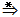αAaβ , a是终结符}。注意，在推导的某一时刻，在A和a之间可能有符号，但如果是这样，它们将推导出ε并消失。如果A是某个句型的最右符号，那么#属于FOLLOW(A)。(注: #是输入串的结束符)

六：FOLLOW集的求法

Follow集合是针对非终结符而言的，Follow(U)所表达的是句型中非终结符U所有可能的后随终结符号的集合，特别地，“#”是识别符号的后随符。注意Follow集合是从开始符号S开始推导。

\1. 直接收取：注意产生式右部的每一个形如“…Ua…”的组合，把a直接收入到Follow(U)中。因a是紧跟在U后的终结符。

2．直接收取：对形如“…UP…”(P是非终结符)的组合，把First§直接收入到Follow(U)中【在这里，如果First（P）中有空字符，那么就要把左部（假设是S）的Follow（S）送入到Follow（U）中。还有就是Follow集中是没有空字符的】。

\3. 直接收取：若S－>…U，即以U结尾，则#∈Follow(U)

4．*反复传送：对形如U－>…P的产生式（其中P是非终结符），应把Follow(U)中的全部内容传送到Follow§中。

另外，比较正式的说明如下：

FIRST集的计算:

首先，为了计算单个文法符号X的FIRST(X)，可以应用下列规则，直到没有终结符或ε可加到某个FIRST集合为止：

\1. 如果X是终结符，则FIRST(X)是 { X }。

\2. 如果X –> ε 是一个产生式，则将ε加到FIRST(X)中。

\3. 如果X是非终结符，且X –> Y1Y2…Yk是一个产生式,其中k≥1。则当某终结符a∈FIRST(Yi),(1<i≤k)且

​ ε∈FIRST(Y1),ε∈FIRST(Y2)…,ε∈FIRST(Yi-1)时，就把a加入到FIRST(X)中去，换句话说就是

​ Y1Y2…Yi-1ε 。如果ε∈FIRST(Yj) 其中j=1,2,…,k，就把ε也加入FIRST(X)中。

知道了如何计算单个文法符号的FIRST集，那么我们就可以计算一组文法符号串的FIRST集。假设这一组文法符号串由X1X2…Xn构成。首先将集合FIRST(X1)中的除ε的终结符号加入FIRST(X1X2…Xn).依次，如果

ε∈FIRST(X1)，那么将集合FIRST(X2)中除ε的终结符号也加入FIRST(X1X2…Xn),依次类推。最后，如果X1,X2,…Xn中每一个文法符号的FIRST集当中都有ε,那么把ε也加入到FIRST(X1X2…Xn)中。

FOLLOW集的计算:

为了计算文法中每一个非终结符X的FOLLOW(X)，应用如下的三条规则，直到没有任何一个终结符能被添加到任何非终结符的FOLLOW集当中为止。

\1. 如果S是文法的开始符号,那么把 添 加 进 F O L L O W ( S ) 中 。 ( 添加进FOLLOW(S)中。( 是输入串的结束符)

\2. 如果有一个产生式A->αBβ，那么将集合FIRST(β)中除ε外的所有元素加入到FOLLOW(B)当中。

\3. 如果有一个产生式 A->αB , 或者A->αBβ且FIRST(β)中包含ε , 那么将集合FOLLOW(A)中的所有元素

​ 加入到集合FOLLOW(B)中。

另一种描述：

计算FIRST集

对每一文法符号X∈V , 计算FIRST(X)
(a) 若X∈VT，则FIRST(X)＝{X}
(b) 若X∈VN，且有产生式 X→ a… , a∈VT ,则 a∈FIRST(X) 即把a加入到FIRSR(X)中

(d) 若X∈VN, 且有产生式X→Y1 Y2 …Yi-1 Yi … Yn,当Y1 Y2 … Yi-1都能推出ε时，
则 FIRST(Y1)－{ε}
FIRST(Y2)－{ε}

FIRST(Yi-1)－{ε}
FIRST(Yi)
都包含在FIRST(X)中。

(e) 当(d)中X→Y1 Y2 … Yn 所有Yj 都可以推出ε,(j=1,2,…n) ,
则 FIRST(Y1)
FIRST(Y2)

FIRST(Yn)
都包含在FIRST(X)中
反复使用上述(b)～(e)步直到每个符号的FIRST集合不再增大为止。

求一个符号串α的FIRST集合

若符号串α∈V*，α=X1 X2 … Xi-1 Xi … Xn,
1.若X1不能推导出ε, 则 FIRST(α)= FIRST(X1)
2.若对任何j (1≤j≤i-1, 2≤i≤n) ε∈FIRST(Xj)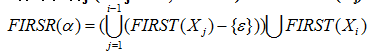3.若对任何j (1≤j≤n) ε∈FIRST(Xj),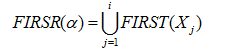七：例子

有如下文法，
S->AaBb|BbBa

A->ε

B->ε
我们求FIRST集。
FIRST(S) = FIRST(AaBb) ∪ FIRST(BbBa)

我们看到

FIRST(A) = ε

FIRST(B) = ε

于是，根据我们的规则，要向下看，
FIRST(a) = a

FIRST(b)= b

没有ε 于是结束，并且在FIRST(AaBb)中不加入ε。
那么我们的结果就是，FIRST(S) = FIRST(AaBb) ∪ FIRST(BbBa) = {A,B}

例：判断该文法是不是LL(1)文法，说明理由

S→ABc

A→a|ε

B→b|ε

First集合求法就是：能由非终结符号推出的所有的开头符号或可能的ε，但要求这个开头符号是终结符号。如此题A可以推导出a和ε，所以FIRST（A）=｛a，ε｝；同理FIRST（B）={b,ε};S可以推导出aBc，还可以推导出bc，还可以推导出c，所以FIRST(S）=｛a，b，c｝。
Follow集合的求法是：紧跟随其后面的终结符号或＃。但文法的识别符号包含＃，在求的时候还要考虑到ε。具体做法是把所有包含你要求的符号的产生式都找出来，再看哪个有用。 Follow（S）=｛＃｝ 如求A的，产生式：S→ABc A→a|ε ，但只有S→ABc 有用。跟随在A后年的终结符号是FIRST（B）=｛b，ε｝，当FIRST（B）的元素为ε时，跟随在A后的符号就是c，所以 Follow（A）=｛b，c｝ 同理Follow（B）=｛c｝。

补充: SELECT集 选择集合

给定上下文无关文法的产生式A→α, A∈VN, α∈V*,
若α不能ε，
SELECT(A→α) = FIRST(α)
若αε，
SELECT(A→α) = (FIRST(α)－{ε})∪ FOLLOW(A)

小结：

​ 因为编译原理对求First集、Follow集和Select集有要求，所以在网上找到了这篇博客，课本上有几个小的知识点补充一下

（1）X->Y1Y2···Yk并且Y1Y2···Yk都能->ε，则FIRST(Yk)∈FIRST(X)

（2）如果A->αBβ，则FIRST(β)中除ε之外的所有符号都在FOLLOW(B)中；

小结：

​ 因为编译原理对求First集、Follow集和Select集有要求，所以在网上找到了这篇博客，课本上有几个小的知识点补充一下

（1）X->Y1Y2···Yk并且Y1Y2···Yk都能->ε，则FIRST(Yk)∈FIRST(X)

（2）如果A->αBβ，则FIRST(β)中除ε之外的所有符号都在FOLLOW(B)中；

​ 如果A->αB，或A->αBβ，且FIRST(β)包含ε，那么FOLLOW(A)∈FOLLOW(B).

展开全文• 最近学习到First集 Follow集 Select集感到比较困难，查询了多篇文章，做下此学习笔记 整合了下面多篇大佬文章，在此做学习笔记记录： first集&follow集&select集. [编译原理]FIRST集合FOLLOW集的介绍和求解....

## 最近学习到First集 Follow集 Select集感到比较困难，查询了多篇文章，做下此学习笔记

整合了下面多篇大佬文章，在此做学习笔记记录：

# 正文开始

一些符号和定义：
一般大写字母表示非终结符，小写字母表示终结符。
文法式一套有限的规则，可以创建无限符合此规则的句子。## First 集

定义：FIRST(α)是α的所有可能推导的开头终结符或可能的ε。
注意区分上面的alpha和a，first(α)表示以α开始符号的集合，α所有可能推导的开头终结符或者式ε.

求解规则：小结：
1、对于终结符而言，FIRST集中的元素只有它本身
2、对于非终结符而言，如果开始符是终结符或者空符号串ε，则加入其FIRST集中；若开始符是非终结符，则要加入它的不含ε的FIRST集，并考虑其为ε时的情况。

技巧：
First一般从下往上找。
如果要找A的First，我们要找A的定义式，即A在左边的式子，看着他的右边来找。

## Follow集

定义：

FOLLOW(A)是所有该文法开始符推导的句型中出现在紧跟A之后的终结符或 “#”。
其中S表示文法的开始符号，非终结符A后面跟着的终结符或‘#’集合，且式紧挨着A的如果文法的开始符号S，把#加入到follow(S)
如果A->αBβ是一个产生式，把first(β){ε}加入到follow(B)中.（first(β){ε}表示把first(β)中去掉ε)
如果A->αB是一个产生式，或A->αBβ是一个产生式且β->ε，则把follow(A)加入到follow(B)中。
**注意，**A->αBβ，α可以是终结符也可以为非终结符也可以为空，β可以为终结符或者非终结符，但是不能为空且B后面要有符号;

求解规则：如S->(L) | aL | LC
找Follow的三种情况：
先在候选式（右边）中找到该非终结符，如L（注意例中只有一个定义，但找Follow要看到所有右边出现该非终结符的）

如果L的右边是终结符， 那么这个终结符加入L的Follow
如果L的右边是非终结符， 那么把这个非终结符的First除去空加到L的Follow中
如果L处在末尾，那么，’->'左边符号的Follow成为L的Follow

小结：
1、FOLLOW集对于非终结符而言，是非终结符的全部后跟符号的集合，如果后跟终结符则加入，如果后跟非终结符，则加入该非终结符的不含空符号串的FIRST集，特别地，文法的识别符的FOLLOW集需要额外的加入‘#’。

技巧
Follow一般从上往下找。
如果要找L的Follow，要从式子的右边找到L，然后来找L的Follow，这与First是不同的。

## SELECT集

定义
给定上下文无关文法的产生式A→α, A∈VN,α∈V*
若α不能推导出ε则：SELECT(A→α)=FIRST(α)
如果α能推导出ε则：SELECT(A→α)=（FIRST(α) –{ε}）∪FOLLOW(A)
需要注意的是，SELECT集是针对产生式而言的。
意义
给定输入的表达式字符串需要根据表达式的字符串中的字符用来寻找正确的产生式，即输入的表达式字串中的字符作为key，产生式作为value，那么这个select集就是构造的map

## 举例①

文法：
S→ABc
A→a|ε
B→b|ε

### First集合求法:

能由非终结符号推出的所有的开头符号或可能的ε，但要求这个开头符号是终结符号。

如此题A可以推导出a和ε，所以FIRST（A）=｛a，ε｝；同理 FIRST（B）={b,ε};S可以推导出aBc，还可以推导出bc，还可以推导出c，所以FIRST(S）=｛a，b，c｝

### Follow集合的求法:

紧跟随其后面的终结符号或＃。但文法的识别符号包含＃，在求的时候还要考虑到ε。

具体做法是把所有包含你要求的符号的产生式都找出来，再看哪个有用。 Follow（S）=｛＃｝
如求A的Follow集 产生式：S→ABc A→a|ε ，但只有S→ABc 有用。跟随在A后的终结符号是FIRST（B）=｛b，ε｝，当FIRST（B）的元素为ε时，跟随在A后的符号就是c，所以 Follow（A）=｛b，c｝ 同理Follow（B）=｛c｝

## 举例②：写出下面文法中所有非终结符的FIRST集

	E → TE’
E’ → +E|ε
T → FT’
T’ → T|ε
F → PF’
F’ → *F’|ε
P  → (E)|a|b|^


规则：FIRST(E’) = {+ , ε}  			规则(1.1)(1.2)
FIRST(F’) = {* , ε}    		 	规则(1.1)(1.2)
FIRST(P)  = {( , a , b , ^}		        规则(1.1)
FIRST(F)  = FIRST(PF’) = FIRST(P)\{ε}= {( , a , b , ^}	规则(1.3)  		FIRST(P)不包含ε
FIRST(T)  = FIRST(FT’) = FIRST(F)\{ε}= {( , a , b , ^}	规则(1.3)  		FIRST(F)不包含ε
FIRST(E)  = FIRST(TE’) = FIRST(T)\{ε}= {( , a , b , ^}	规则(1.3)  		FIRST(T)不包含ε
FIRST(T’) = {FIRST(T)\{ε}} ∪ {ε} = {( , a , b , ^ ，ε}	规则(1.2)(1.3)	FIRST(T)不包含ε


## 举例③：写出下面文法中求FIRST(S)，FIRST(A)，FIRST(B)

	G: S → BA
A → BS|d
B → aA|bS|c


FIRST(A) = {FIRST(B)/{ε}}∪{d} = {a, b, c, d}
FIRST(S) = {FIRST(B)/{ε}} = {a, b, c}
FIRST(B)={a, b, c}

## 举例④：写出下面文法中所有非终结符的FIRST集

	E → TE’
E’ → +E|ε
T → Fd
F → PF’
F’ → *F’|ε
P → (E)|a|b|ε


答案：

	FIRST(E)  = FIRST(TE’) = FIRST(T) = {( , a, b, *, d}
FIRST(E’) = {+, ε}
FIRST(T)  = FIRST(Fd)
= {FIRST(F)\{ε}}∪{d}
= {(, a, b, *, d}
FIRST(F)  = FIRST(PF’)
= {FIRST(P)\{ε}}∪{FIRST(F’)\{ε}}∪{ε}
= {(, a, b, *, ε}
FIRST(F’) = {*, ε}
FIRST(P)  = {(, a, b, ε}


## 举例⑤：写出下面文法中所有非终结符的FOLLOW集

     G: E → TE’
E’ → +TE’|ε
T → FT’
T’ → *FT’|ε
F → (E)|i
已知：
FIRST(E) = FIRST(T) = FIRST(F) = {(, i}
FIRST(E’) = {+, ε} 	FIRST(T’) = {*, ε}


规则：1、由规则(2.1)，对文法开始符E，置#于FOLLOW(E)中
# ∈ FOLLOW(E)
2、E在产生式F → (E)|i 的右部出现，由规则(2.2)，结合步骤1
FOLLOW(E) = {#，)}
3、E’在产生式E → TE’和 E’ → +TE’|ε的右部出现，由规则(2.3)
FOLLOW(E’) = FOLLOW(E)∪FOLLOW(E’) = {#，)}
4、T在产生式 E → TE’和E’ → +TE’|ε 的右部出现，由规则(2.2)，再由规则(2.3)
FOLLOW(T) = {FIRST(E’)/{ε}}∪FOLLOW(E)∪FOLLOW(E’) = {+，#，)}
5、T’在产生式T → FT’ 和T’ → *FT’|ε 的右部出现，由规则(2.3)
FOLLOW(T’) = FOLLOW(T)∪FOLLOW(T’) = {+，#，)}
6、F在产生式T → FT’ 和T’ → FT’|ε的右部出现，由规则(2.2)，再由规则(2.3)
FOLLOW(F) = {FIRST(T’)/{ε}}∪FOLLOW(T)∪FOLLOW(T’) = {
，+，#，)}

## 举例⑥：求SELECT集

G(E):E->TE'
E'->+TE' | ε
T->FT'
T'->*FT' | ε
F->(E) | i

对应的first集合：
first(E) = {i, (}
first(T) = {i, (}
first(E') = {+, ε}
first(T') = {*, ε}
first(F) = {(, i}

follow(E) = {#, )} // E作为开始符，且在F->(E) | i 产生式中，E后存在 ) 符号
follow(E') = {#, )} // 根据规则 A->αB，则E->TE'将follow(E)加入到follow(E')
follow(T) = {+, #, )} // 有E->TE'，根据A->αBβ规则，有α为空，E'->ε，此时将follow(E)加入follow(T)，根据E'->+TE' | ε将first(+)\{ε}加入follow(T)
follow(T') = {+, #, )} // T->FT'将follow(T)加入
follow(F) = {(*,+,#,)} // T->FT'，根据A->αBβ，α为空，T'->ε，将follow(T)加入;T'->*FT' | ε，根据A->αBβ，将first(T') / {ε} 加入
上面已经计算过这个例子的first和follow集答案：

select(E->TE') = {i, (} // T无法推出ε，所以取first(T)
select(E'->+TE') = {+} // 直接取first(+)
select(E'->ε) = {#, )} // 注意候选式要分开计算，因为是ε所以取follow(E')
select(T) = {(,i} // first(F)
select(T'->*FT') = {*} // first(*)
select(T'->ε） = {+, #, )} // follow(T')
select(F->(E)) = {(} // first(()
select(F->i) = {i} // first(i)

展开全文编译器
• . . . . .下载可编辑. 构造预测分析表 源程序 #include<stdlib.h> #include<stdio.h> #include<string.h> /*/ int count=0; /*分解的产生式的个数*/ int number; /*所有终结符和非终结符的总数*/ char start;...
• 编译实验中求一文法的first集和follow集
• ( 编译原理JAVA求First集Follow集
• 编译原理课程设计First集和Follow集生成算法模拟 【问题描述】 设计一个由正规文法生成First集和Follow集并进行简化的算法动态模拟 【基本要求】 动态模拟算法的基本功能是： （1） 输入一个文法G； （2） 输出由...
• 对文法中的非终结符，求first集和follow集
• 为了方便自顶向下语法分析，需要求文法对应的first集，follow集，以及select集。本文主要分为两部分，一个是求法解析，还有一个例子详解：第一部分是求法解析将对first集，follow集，select集分为三种讲解方法①定义...编译原理 follow集 select集
• LL（1）分析法 消除左递归，提取左因子，求解FIRST集，求解FOLLOW集python
• First集算法描述 1.若X->a…，则将终结符a放入First（X）中 2.若X->ε，则将ε放入First（X）中 3.若有X->Y1Y2Y3…Yk，则 （1）把First（Y1）去掉 ε后加入First（X） （2）如果First（Y1）包含ε，则...编译原理 c++
• 编译原理的FIRST集和FOLLOW集~~有兴趣的可以看一下，有漏了一个条件，不过注明出来了~~
• 2021年编译原理课程设计求follow集、first集、predict集 可运行，简洁，有详细注释
• //所有非终结符的first集 string f =""; //follow集 //将文件中的文法转换成产生式 void fun1(string str){ exp e; e.ter = str; int i = 3; for(int k = i;k ();k++){ if(str[k] == '|'){ e....C++ follow集 编译原理
• 编译原理之first集 & follow集 & select集 详解 一、first集，follow集，以及select集求法解析 二、例子详解 1、关于first集： 2、关于follow集： 3、关于select集： 叮嘟！这里是小啊呜的学习课程资料整理。好记性...编译器
• First集合可分如下几种情况:1、单个符号的First集合：单个终结符的First集合就是它自己。2、单个非终结符的First集合： A-->a…产生式右部以终结符开头，根据定义，这种情况下显然可以看出a属于First(A)。.
• 一、FIRST集 FIRST(A)为A的开始符或者首符号集。 1、定义： 设G=(VT，VN，S，P)是上下文无关文法 ，FIRST(α)={a|α能推导出aβ,a∈VT，α,β∈V*} 特别的，若α能推导出ε,则规定ε∈FIRST(α)． 2、根据定义求解...
• 文章目录FIRST集的求法FOLLOW集的计算生成预期分析表算法例题 FIRST集的求法 FIRST集是一个文法符号串所可能推导出的符号串的第一个终结符的集合 对于文法G的任一符号串α\alphaα = x1x2…xnx_{1}x_{2} \ldots x_{n...编译原理 FOLLOW集
• 编译原理 求first集与follow集 c++ 题目： 输入任意的上下文无关文法,输出所输入的上下文无关文法一切非终结符的first集合和follow集合
• 利用vs中的c语言完成编译原理的follow集合first集的算法实现
• #输入文法求First集和Follow集 #要求大写字母表示非终结符，小写字母表示终结符 #最后一个产生式以$结尾或者输入$表示输入结束 #默认第一个产生式的→左边为起始符号 def inputGrammer(): #接收文法输入的函数 ...python 编译原理 Follow集...